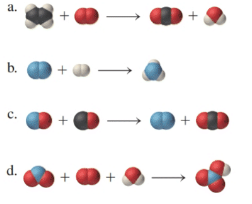# For each chemical reaction, draw in the missing molecules necessary to balance their equations. Carbon atoms are black, hydrogen atoms are white, oxygen atoms are red, and nitrogen atoms are blue.### Chemistry In Focus

7th Edition
Tro + 1 other
Publisher: Cengage Learning,
ISBN: 9781337399692

#### Solutions

Chapter
Section### Chemistry In Focus

7th Edition
Tro + 1 other
Publisher: Cengage Learning,
ISBN: 9781337399692
Chapter 4, Problem 63E
Textbook Problem
545 views

## For each chemical reaction, draw in the missing molecules necessary to balance their equations. Carbon atoms are black, hydrogen atoms are white, oxygen atoms are red, and nitrogen atoms are blue.Interpretation Introduction

Interpretation:

The chemical reactions are to be balanced for the given molecular model reactions, where carbon atoms are black, hydrogen atoms are white, oxygen atoms are red, and nitrogen atoms are blue.

Concept Introduction:

A chemical equation is considered to be the symbolic depiction of a chemical reaction, which contains symbols and formula.

In a chemical reaction, the starting substances on the left side are known as reactants, while the substances on the right side are known as products.

The chemical formula represents the precise number of atoms in the molecule, but not their structural arrangement

The molecular model represents the molecules in a three-dimensional configuration.

### Explanation of Solution

a)

In the given molecular model, the reaction can be represented in a chemical reaction as follows:

C2H4+O2CO2+H2O

C atom is balanced by multiplying with a coefficient 2 in CO2 as follows:

C2H4+O22CO2+H2O

In the next step, the O atom is balanced by the multiplying with a coefficient 3 in O2 as follows:

C2H4+3O22CO2+H2O

Now, in the final step, the H atom is balanced by the multiplying with a coefficient 2 in H2O as follows:

C2H4+3O22CO2+2H2O

b)

In the given molecular model, the reaction can be represented in a chemical reaction as follows:

N2+H2NH3

N atom is balanced by multiplying with a coefficient 2 in NH3 as follows:

N2+H22NH3

In the next step, the H atom is balanced by the multiplying with a coefficient 3 in H2 as follows:

N2+3H22NH3

c)

In the given molecular model, the reaction can be represented in a chemical reaction as follows:

NO+CON2+CO2

### Still sussing out bartleby?

Check out a sample textbook solution.

See a sample solution

#### The Solution to Your Study Problems

Bartleby provides explanations to thousands of textbook problems written by our experts, many with advanced degrees!

Get Started

Find more solutions based on key concepts
How do eating disorders affect health?

Understanding Nutrition (MindTap Course List)

A built environment can support physical activity with a. safe biking and walking lanes. b. public parks. c. fr...

Nutrition: Concepts and Controversies - Standalone book (MindTap Course List)

Is the urea cycle an energy-producing or an energy consuming pathway?

Introduction to General, Organic and Biochemistry

Name the following alkanes.

Chemistry: Principles and Reactions

What two nonstandard amino acids are present in collagen?

General, Organic, and Biological Chemistry

A hydrometer is an instrument used to determine liquid density. A simple one is sketched in Figure P14.16. The ...

Physics for Scientists and Engineers, Technology Update (No access codes included)